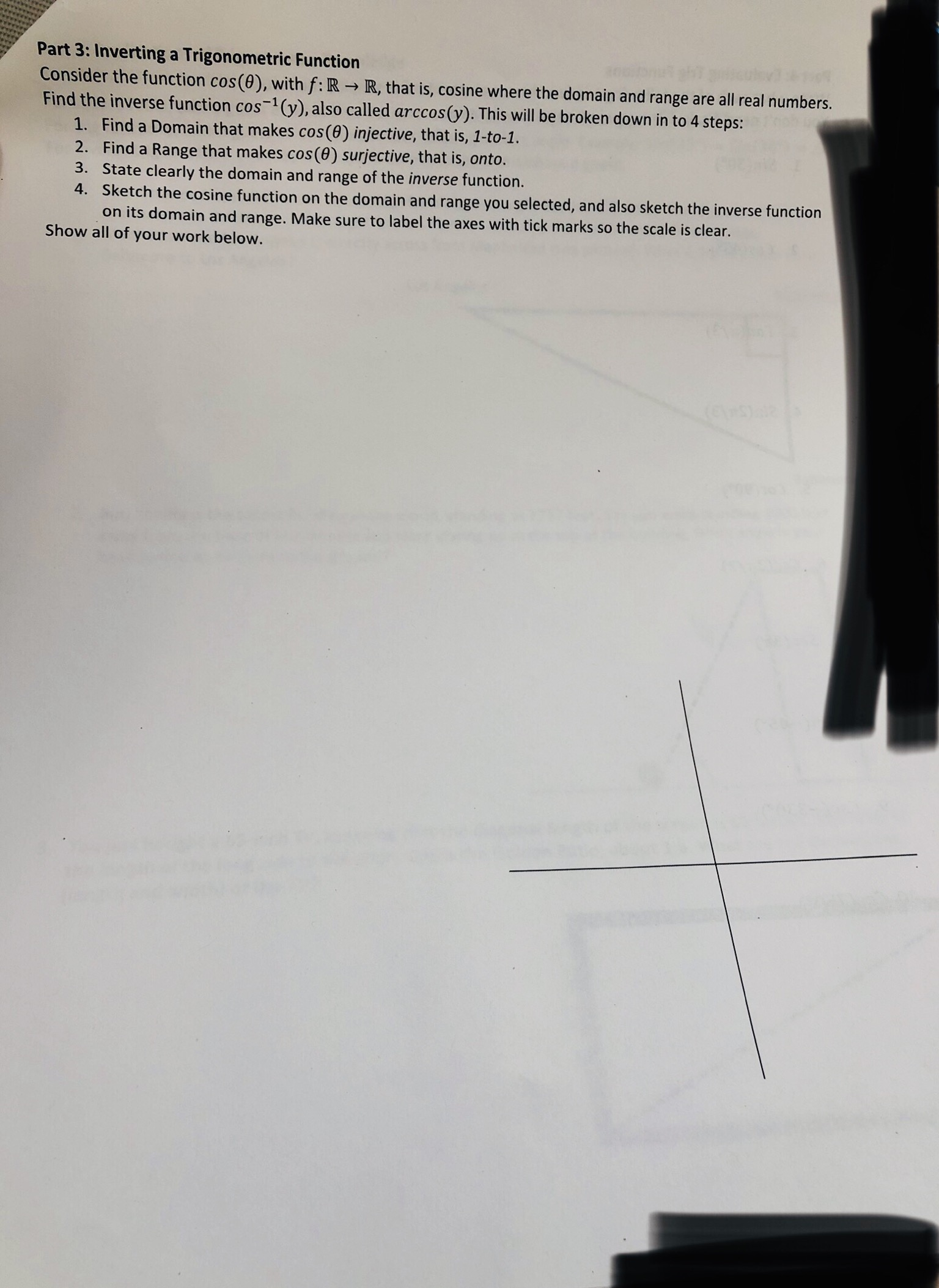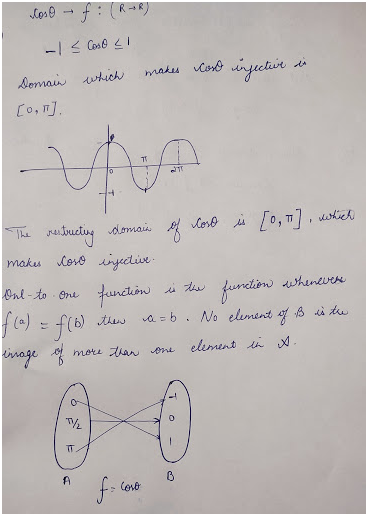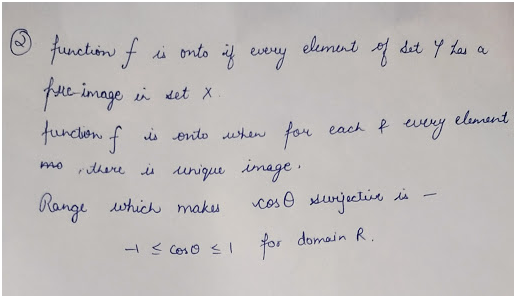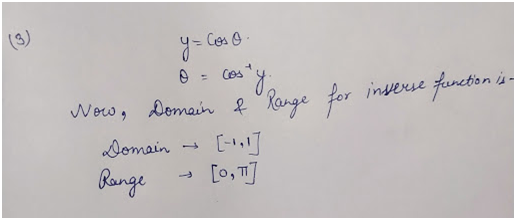# Part 3: Inverting a Trigonometric FunctionConsider the function cos(0), with f: R R, that is, cosine where the domain and range are all real numbers.Find the inverse function cos-1(y), also called arccos(y). This will be broken down in to 4 steps:1. Find a Domain that makes cos(0) injective, that is, 1-to-1.2. Find a Range that makes cos(0) surjective, that is, onto.3. State clearly the domain and range of the inverse function.4. Sketch the cosine function on the domain and range you selected, and also sketch the inverse functionon its domain and range. Make sure to label the axes with tick marks so the scale is clear.Show all of your work below.

Question
329 viewshelp_outlineImage TranscriptionclosePart 3: Inverting a Trigonometric Function Consider the function cos(0), with f: R R, that is, cosine where the domain and range are all real numbers. Find the inverse function cos-1(y), also called arccos(y). This will be broken down in to 4 steps: 1. Find a Domain that makes cos(0) injective, that is, 1-to-1. 2. Find a Range that makes cos(0) surjective, that is, onto. 3. State clearly the domain and range of the inverse function. 4. Sketch the cosine function on the domain and range you selected, and also sketch the inverse function on its domain and range. Make sure to label the axes with tick marks so the scale is clear. Show all of your work below. fullscreen
check_circle

Step 1

1) Find the domain-Step 2

2) Find the range:Step 3

3) Find the Domain and range of inverse function:...

### Want to see the full answer?

See Solution

#### Want to see this answer and more?

Solutions are written by subject experts who are available 24/7. Questions are typically answered within 1 hour.*

See Solution
*Response times may vary by subject and question.
Tagged in